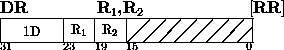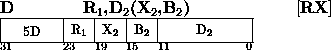Next: exclusive OR Up: Instructions Previous: Compare Logical

### DivideThe dividend (first operand) is divided by the divisor (second operand) and replaced by the remainder and the quotient.

The dividend is a 64-bit signed integer and occupies the even-odd pair of registers specified by the R1 field of the instruction. A specification exception occurs when R1 is odd. A 32-bit signed remainder and a 32-bit signed quotient replace the dividend in the even-numbered and odd-numbered registers, respectively. The divisor is a 32-bit signed integer.

The sign of the quotient is determined by the rules of algebra. The remainder has the same six as the dividend, except that a zero quotient or a zero remainder is always positive. When the relative magnitude of dividend and divisor is such that the quotient cannot be expressed by a 32-bit signed integer, a fixed-point divide exception is recognized (a program interruption occurs, no division takes place, and the dividend remains unchanged in the general registers).

Condition Code:
The code remains unchanged.

Program Exceptions:
Access (fetch, operand 2 of D only) Specification
Fixed-Point DivideNext: exclusive OR Up: Instructions Previous: Compare Logical

A.Daviel,TRIUMF